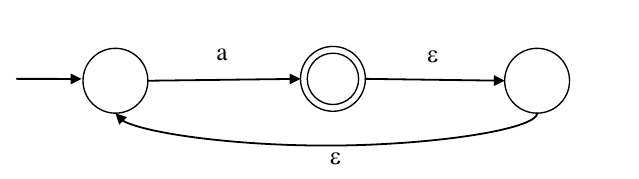6.1k views

What is the complement of the language accepted by the NFA shown below?
Assume $\Sigma = \{a\}$ and $\epsilon$ is the empty string.1. $\phi$
2. $\{\epsilon\}$
3. $a^*$
4. $\{a , \epsilon\}$

edited | 6.1k views
0
What will be the NFA for the complement of the lang?

All state transition arrows will be reversed and non-final states will be final and vice-versa. Is it correct?
+2
arrows will not be reversed.....
0

Assume $\sum=\{a\}$ and $\epsilon$ is the empty string.$L=\left\{a^{+}\right\}$

$(1)$ What is the complement of the language accepted by the $NFA?$

$\overline{L}=\left\{\epsilon\right\}$
$(2)$ What is the language which accepts complement of $NFA?$$L_{1}=\left\{\epsilon,(a+\epsilon)^{+}\right\}$

Please correct me if i'm wrong$?$

+8
No,  it is not correct as it is epsilon-nfa. In nfa,   we do not get a complement by complementing the state diagram. You have to complement the language.  So,  language accepted here is L={a+}.

So,  it's complement would be L'={€}
0
which one is not correct??
0

@Lakshman Patel RJIT

why the answer is not $a^{*}??$

If we draw complement diagram , isnot it coming $a^{*}??$

I mean according to this diagram ,what it means?+3

@srestha ma'amIt is $\epsilon$-NFA

And when we just toggled the state, we can't get the complement of $\epsilon$-NFA.

In my above comment $2^{nd}$ statement is not correct.It is not a complement of above $\epsilon$-NFA. Because it also accepts $L=\{a^{+}\}.$

---------------------------------------------------------------------------------$L=\{a,aa,aaa,...\}=\{a^{+}\}$

We can draw the DFA for this.$L=\{a^{+}\}$

Now, we can complement the DFA$L=\{\epsilon\}$

Reference:

0

@Lakshman Patel RJIT

yes, that I know, but what this diagram accepts??

is it $a^{*}$ or $\epsilon ??$0

Ma'am

it will give $L=\{\epsilon,(a+\epsilon)^{+}\}=\{\epsilon,a^{+}\} = \{a^{\ast}\}$

and below automata is also giving the same output+1
So, ur answer is not matching, hence not correct. right??

We can conclude that , we cannot do complement of NFA. Complement only possible for DFA.

right??
0
Yes
+1

1. Complement of language of a given NFA $\neq$  Language accepted by Complement of that given NFA.

2. Complement of language of a given DFA $=$  Language accepted by Complement of that given DFA.

0
I think you are right.
+1

@MRINMOY_HALDER

Complement of language of a given NFA ≠  Language accepted by Complement of that given NFA.

2. Complement of language of a given DFA =  Language accepted by Complement of that given DFA.

Can u explain these lines ?  Where u got these line?

0
"Complement of language of a given NFA ≠≠  Language accepted by Complement of that given NFA. "

I think it is may or may not be equal. Please verify.

The language being accepted is $a^+$. So, complement of the language is $\{\epsilon\}$.
by
selected by
+3
How will aa be produced here ?  after one a it goes to final state . What hapens on reading the 2nd 'a'  ?
+19
@gokou from final state using epsilon we can again come back to start state..and the process repeats!!
0
Is this true?: If L = $\phi$ then L* = $\{\epsilon\}$
0
@habed yes true
0
Sir, I have the same query. If in complementation, arrows are not reversed, then there are always epsilon paths from the non-final state(middle state) to the final states. So language accepted should be a*.

But again, your solution also seems very right.  I fail to understand what's going on.

It would be really helpful if you can clarify this doubt.
+3

In Complementation, arrows are not reversed but final states are exchanged so that change the whole language .

Example: make DFA of a* now if you complete the DFA then there is no Final state i.e. accept Nothing.

Take another language a*b* make DFA of it and complement it , Then you get the idea.

0
Plss someone clarify why answer is not a*. According to me suppose we give input string 'aaa'. Then using epsilon transitions we can reach the final state. overall it can generate a*.

Plss help.
+1
@mbisht complement rule is not applicable on NFA it is applicable on dfa so first convert it into dfa then come to point .....u should read prashant sir comment
0
Thankss...I got it.
0

from final state using epsilon we can again come back to start state..and the process repeats!!

using the same logic, even in complement of NFA this should work.

Plz help

0
@Arjun sir how dfa will look like for this nfa ? Can we just remove epsilon transitions and can consider it as dfa? Or shall we use nfa to dfa conversion method?
+1

When we complement a NFA does the language always get complemented ? plz explain @arjun sir0
sir complement of finite automata only work for DFA , not for NFA . can you plz explain ?
+3

$1.\ \varepsilon- NFA\ to\ NFA$$2.\ NFA\ to\ DFA$$3.\ Compliment$$Ans: \varepsilon$

NFA accepts the language L=a+ and ∑={a}

the complement of L=∑*- a+=a*-a+={∊}

0
Complement does not work with NFA always. I don't think this approach is correct even though it works for this example.
+1

@tusharp he did not make the complement of NFA, he made the complement of language which always works

Ans.the language is a+ .....  compliment is {$\varepsilon$}

edited
one more way is to convert this epsilon nfa to nfa and then take complement of the language but the only careful point is that the question has asked about the langauge formed by complement of the language accpeted by such nfa.

in nfa complementation doesnt work the same way that dfa does. so "complement of language accepted by nfa" and "complement of the machine" are two different things in case of nfa
+1 vote
Here the important is: "complement of the language accepted by the NFA".

The Σ= {a} and the given NFA accepts the strings {a, aa, aaa, aaaa, ……….} i.e. the language accepted by the NFA can be represented by the regular expression: {a+}
Hence the complement of language is: {a* − a+} = {ϵ}

option c is rightedited

L(M)=a+

L'(M)=sigma*-L(M) = {€}